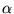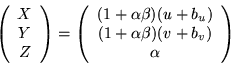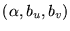Next: Experiments Up: The Proposed Nonlinear Recursive Previous: The EKF Implementation

## Biased Measurements

We turn attention here to the issue of biased measurement noise in the EKF and how it relates to representation of object structure.

We have assumed that features are identified in the first frame and that measurements are obtained by comparing new images to the previous images and that our measurements are zero-mean or very close to zero-mean. This thinking leads to thedescription of structure given earlier in which the single unknown depth for each feature fully describes structure. These parameters can be computed very effectively using the EKF, which assumes zero-mean measurements.

It is common to use Kalman filters even when measurements are not truly zero-mean. Good results can be obtained if the biases are small. However, if the measurements are biased a great deal, results may be inaccurate. In the case of large biases, the biases are observable in the measurements and can therefore be estimated by augmenting the state vector with additional parameters representing the biases of the measurements. In this way, the Kalman filter can in principle be used to estimate biases in all the measurements.

However, there is a tradeoff between the accuracy that might be gained by estimating bias and the stability of the filter, which is reduced when the state vector is enlarged. When the biases are large, i.e. compared to the standard deviation of the noise, they can be estimated and can contribute to increased accuracy. But if the biases are small, they cannot be accurately estimated and they do not affect accuracy much. Thus, it is only worth augmenting the state vector to account for biases when the biases are known to be significant relative to the noise variance.

In the SfM problem, augmenting the state vector to account for bias adds two additional parameters per feature. This results in a geometry representation having a total of 7+3N parameters. Although we do not recommend this level of state augmentation, it is interesting because it can be related to the large state vector used in [10,42] and others, where each structure point is represented using three free parameters (X,Y,Z).

If we add noise bias parameters (bu,bv), Equation 14 can be written(20)

This relation is invertible so the representations are analytically equivalent. However, geometrically theparameterization is more elucidating than (X,Y,Z) because it parameterizes structure along axes physically relevant to the measurement process. Thus, it allows us to more effectively tune the filter, ultimately reducing the dimensionality of the state space quite significantly.

It is clear that, in general, uncertainty intrivializes uncertainty in the direction of the biases. By using initial error variance onthat is high in comparison to the error variances on (bu,bv), the state space is essentially reduced because the system responds more stiffly in the direction of the biases, favoring instead to correct the depths. In the limit (zero-mean-error tracking) the biases can be removed completely, resulting in the strictly lower dimensional formulation that we typically use in this paper. Our experimental results demonstrate that bias is indeed a second-order effect and is justifiably ignored in most cases.Next: Experiments Up: The Proposed Nonlinear Recursive Previous: The EKF Implementation

1999-05-17